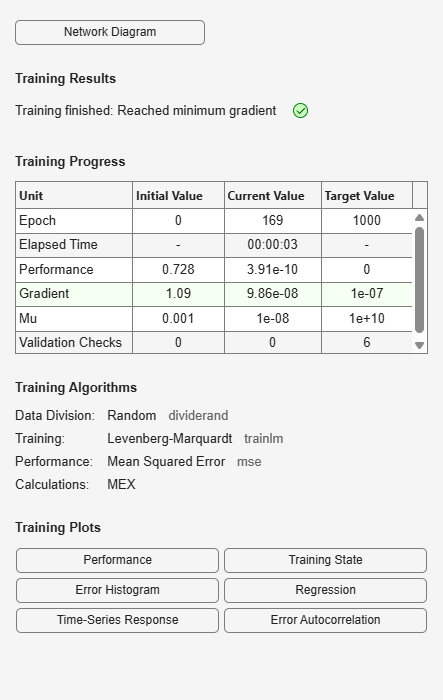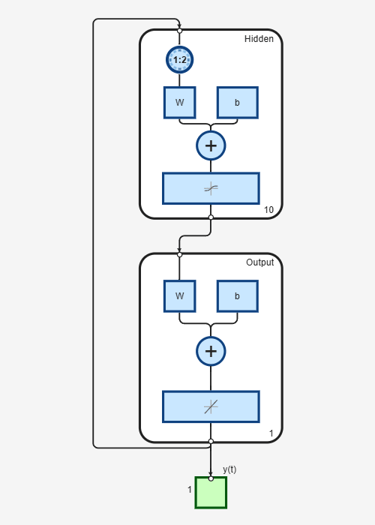# narnet

Nonlinear autoregressive neural network

## Syntax

``narnet(feedbackDelays,hiddenSizes,feedbackMode,trainFcn)``

## Description

example

````narnet(feedbackDelays,hiddenSizes,feedbackMode,trainFcn)` takes these arguments: Row vector of increasing 0 or positive feedback delays, `feedbackDelays`Row vector of one or more hidden layer sizes, `hiddenSizes`Type of feedback, `feedbackMode`Training function, `trainFcn` and returns a NAR neural network. You can train NAR (nonlinear autoregressive) neural networks to predict a time series from the past values of that series.```

## Examples

collapse all

Train a nonlinear autoregressive (NAR) neural network and predict on new time series data. Predicting a sequence of values in a time series is also known as multistep prediction. Closed-loop networks can perform multistep predictions. When external feedback is missing, closed-loop networks can continue to predict by using internal feedback. In NAR prediction, the future values of a time series are predicted only from past values of that series.

Load the simple time series prediction data.

`T = simplenar_dataset;`

Create a NAR network. Define the feedback delays and size of the hidden layers.

`net = narnet(1:2,10);`

Prepare the time series data using `preparets`. This function automatically shifts input and target time series by the number of steps needed to fill the initial input and layer delay states.

`[Xs,Xi,Ai,Ts] = preparets(net,{},{},T);`

A recommended practice is to fully create the network in an open loop, and then transform the network to a closed loop for multistep-ahead prediction. Then, the closed-loop network can predict as many future values as you want. If you simulate the neural network in closed-loop mode only, the network can perform as many predictions as the number of time steps in the input series.

Train the NAR network. The `train` function trains the network in an open loop (series-parallel architecture), including the validation and testing steps.

`net = train(net,Xs,Ts,Xi,Ai);`Display the trained network.

`view(net)`Calculate the network output `Y`, final input states `Xf`, and final layer states `Af` of the open-loop network from the network input `Xs`, initial input states `Xi`, and initial layer states `Ai`.

`[Y,Xf,Af] = net(Xs,Xi,Ai);`

Calculate the network performance.

`perf = perform(net,Ts,Y)`
```perf = 1.0100e-09 ```

To predict the output for the next 20 time steps, first simulate the network in closed-loop mode. The final input states `Xf` and layer states `Af` of the open-loop network `net` become the initial input states `Xic` and layer states `Aic` of the closed-loop network `netc`.

`[netc,Xic,Aic] = closeloop(net,Xf,Af);`

Display the closed-loop network. The network has only one input. In closed-loop mode, this input connects to the output. A direct delayed output connection replaces the delayed target input.

`view(netc)`To simulate the network 20 time steps ahead, input an empty cell array of length 20. The network requires only the initial conditions given in `Xic` and `Aic`.

`Yc = netc(cell(0,20),Xic,Aic)`
```Yc=1×20 cell array Columns 1 through 5 {[0.8346]} {[0.3329]} {[0.9084]} {[1.0000]} {[0.3190]} Columns 6 through 10 {[0.7329]} {[0.9801]} {[0.6409]} {[0.5146]} {[0.9746]} Columns 11 through 15 {[0.9077]} {[0.2807]} {[0.8651]} {[0.9897]} {[0.4093]} Columns 16 through 20 {[0.6838]} {[0.9976]} {[0.7007]} {[0.4311]} {[0.9660]} ```

## Input Arguments

collapse all

Zero or positive feedback delays, specified as an increasing row vector.

Sizes of the hidden layers, specified as a row vector of one or more elements.

Type of feedback, specified as either `'open'`, `'closed'`, or `'none'`.

Training function name, specified as one of the following.

Training FunctionAlgorithm
`'trainlm'`

Levenberg-Marquardt

`'trainbr'`

Bayesian Regularization

`'trainbfg'`

BFGS Quasi-Newton

`'trainrp'`

Resilient Backpropagation

`'trainscg'`

`'traincgb'`

`'traincgf'`

`'traincgp'`

`'trainoss'`

One Step Secant

`'traingdx'`

`'traingdm'`

`'traingd'`

Example: For example, you can specify the variable learning rate gradient descent algorithm as the training algorithm as follows: `'traingdx'`

For more information on the training functions, see Train and Apply Multilayer Shallow Neural Networks and Choose a Multilayer Neural Network Training Function.

Data Types: `char`

## Version History

Introduced in R2010b